시간 제한 메모리 제한 제출 정답 맞은 사람 정답 비율
2 초 512 MB 0 0 0 0.000%

## 문제

In mathematics, some plain words have special meanings. The word "net" is one of such technical terms. In mathematics, the word "net" is sometimes used to mean a plane shape which can be folded into some solid shape.

The following are a solid shape (Figure 1) and one of its net (Figure 2).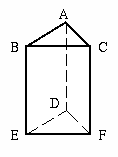Figure 1: a prism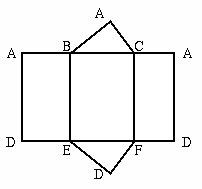Figure 2: a net of a prism

Nets corresponding to a solid shape are not unique. For example, Figure 3 shows three of the nets of a cube.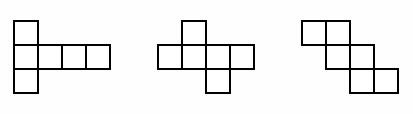Figure 3: examples of nets of a cube

In this problem, we consider nets of dice. The definition of a die is as follows.

1. A die is a cube, each face marked with a number between one and six.
2. Numbers on faces of a die are different from each other.
3. The sum of two numbers on the opposite faces is always 7.

Usually, a die is used in pair with another die. The plural form of the word "die" is "dice".

Some examples of proper nets of dice are shown in Figure 4, and those of improper ones are shown in Figure 5.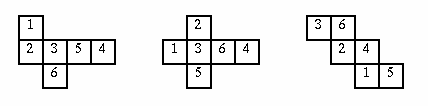Figure 4: examples of proper nets of dice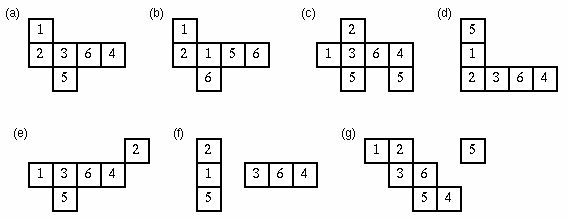Figure 5: examples of improper nets

The reasons why each example in Figure 5 is improper are as follows.

1. The sum of two numbers on the opposite faces is not always 7.
2. Some faces are marked with the same number.
3. This is not a net of a cube. Some faces overlap each other.
4. This is not a net of a cube. Some faces overlap each other and one face of a cube is not covered.
5. This is not a net of a cube. The plane shape is cut off into two parts. The face marked with '2' is isolated.
6. This is not a net of a cube. The plane shape is cut off into two parts.
7. There is an extra face marked with '5'.

Notice that there are two kinds of dice. For example, the solid shapes formed from the first two examples in Figure 4 are mirror images of each other.

Any net of a die can be expressed on a sheet of 5x5 mesh like the one in Figure 6. In the figure, gray squares are the parts to be cut off. When we represent the sheet of mesh by numbers as in Figure 7, squares cut off are marked with zeros.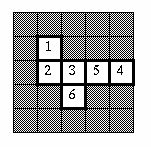Figure 6: 5x5 mesh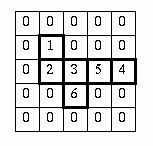Figure 7: representation by numbers

Your job is to write a program which tells the proper nets of a die from the improper ones automatically.

## 입력

The input consists of multiple sheets of 5x5 mesh.

N
Mesh0
Mesh1
...
MeshN-1


N is the number of sheets of mesh. Each Meshi gives a sheet of mesh on which a net of a die is expressed. Meshi is in the following format.

F00 F01 F02 F03 F04
F10 F11 F12 F13 F14
F20 F21 F22 F23 F24
F30 F31 F32 F33 F34
F40 F41 F42 F43 F44

Each Fij is an integer between 0 and 6. They are separated by a space character.

## 출력

For each Meshi, the truth value, true or false, should be output, each in a separate line. When the net of a die expressed on the Meshi is proper, output "true". Otherwise, output "false".

## 예제 입력 1

6
0 0 0 0 0
0 0 0 0 6
0 2 4 5 3
0 0 1 0 0
0 0 0 0 0
0 0 3 0 0
0 0 2 0 0
0 0 4 1 0
0 0 0 5 0
0 0 0 6 0
0 0 0 3 0
0 0 2 5 0
0 4 1 0 0
0 0 6 0 0
0 0 0 0 0
0 6 2 0 0
0 0 4 0 0
0 1 5 0 0
0 0 3 0 0
0 0 0 0 0
0 0 0 0 6
0 2 4 5 3
0 0 1 0 0
0 0 0 0 0
0 0 0 1 0
0 0 0 0 6
0 2 4 5 3
0 0 0 0 0
0 0 0 0 0
0 0 0 1 0


## 예제 출력 1

true
true
false
false
false
false